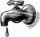School

There are 150 pupils in grade 5 . 2/3 of it are female. By what fractions are the males?

Result

f =  0.333

Solution:Leave us a comment of this math problem and its solution (i.e. if it is still somewhat unclear...):Be the first to comment!To solve this verbal math problem are needed these knowledge from mathematics:

Need help calculate sum, simplify or multiply fractions? Try our fraction calculator.

Next similar examples:

1. Flood waterFlood waters in some US village meant that the homes had to evacuate 364 people. 50 of them stayed at elementary schools, 59 them slept with their friends and others went to relatives. How many people have gone to relatives?
2. ZdeněkZdeněk picked up 15 l of water from a 100-liter full-water barrel. Write a fraction of what part of Zdeněk's water he picked.
3. CakesOn the bowl were a few cakes. Jane ate one-third of them, Dana ate a quarter of those cakes that remained. a) What part (of the original number of cakes) Dana ate? b) At least how many cakes could be (initially) on thebowl?
4. In fractionsAn ant climbs 2/5 of the pole on the first hour and climbs 1/4 of the pole on the next hour. What part of the pole does the ant climb in two hours?
5. SubtractionTest what do you know about the subtraction of two numbers: a) make a difference if the minuend 4,307,288 and subtrahend is 472008 b) minuend is 4000 more than subtrahend. What's the difference? c) the difference is 38900 less than the minuend. What is th
6. Fraction and a decimalWrite as a fraction and a decimal. One and two plus three and five hundredths
7. Lengths of the poolMiguel swam 6 lengths of the pool. Mat swam 3 times as far as Miguel. Lionel swam 1/3 as far as Miguel. How many lengths did mat swim?
8. Mixed2improperWrite the mixed number as an improper fraction. 166 2/3
9. Fraction to decimalWrite the fraction 3/22 as a decimal.
10. Pounds3 pounds subtract 1/3 of a pound.
11. Wood 11Father has 12 1/5 meters long wood. Then I cut the wood into two pieces. One part is 7 3/5 meters long. Calculate the length of the other wood?
12. Roses and tulipsAt the florist are 50 tulips and 5 times less roses. How many flowers are in flower shop?
13. Cakes1/3 poppy cake, 1/3 apple, 15 pieces of cheese. How many are totally cakes?
14. The tapFor one day flows 148 l of water out of the tap. How much water will flow out for 3/4 day?
15. Mixed numbersRewrite mixed numbers so the fractions have the same denominator: 5 1/5 - 2 2/3
16. 3 children3 children had to divide 4 pounds is candy. How much candy did each child get?
17. Addition of Roman numbersAdded together and write as decimal number: LXVII + MLXIV